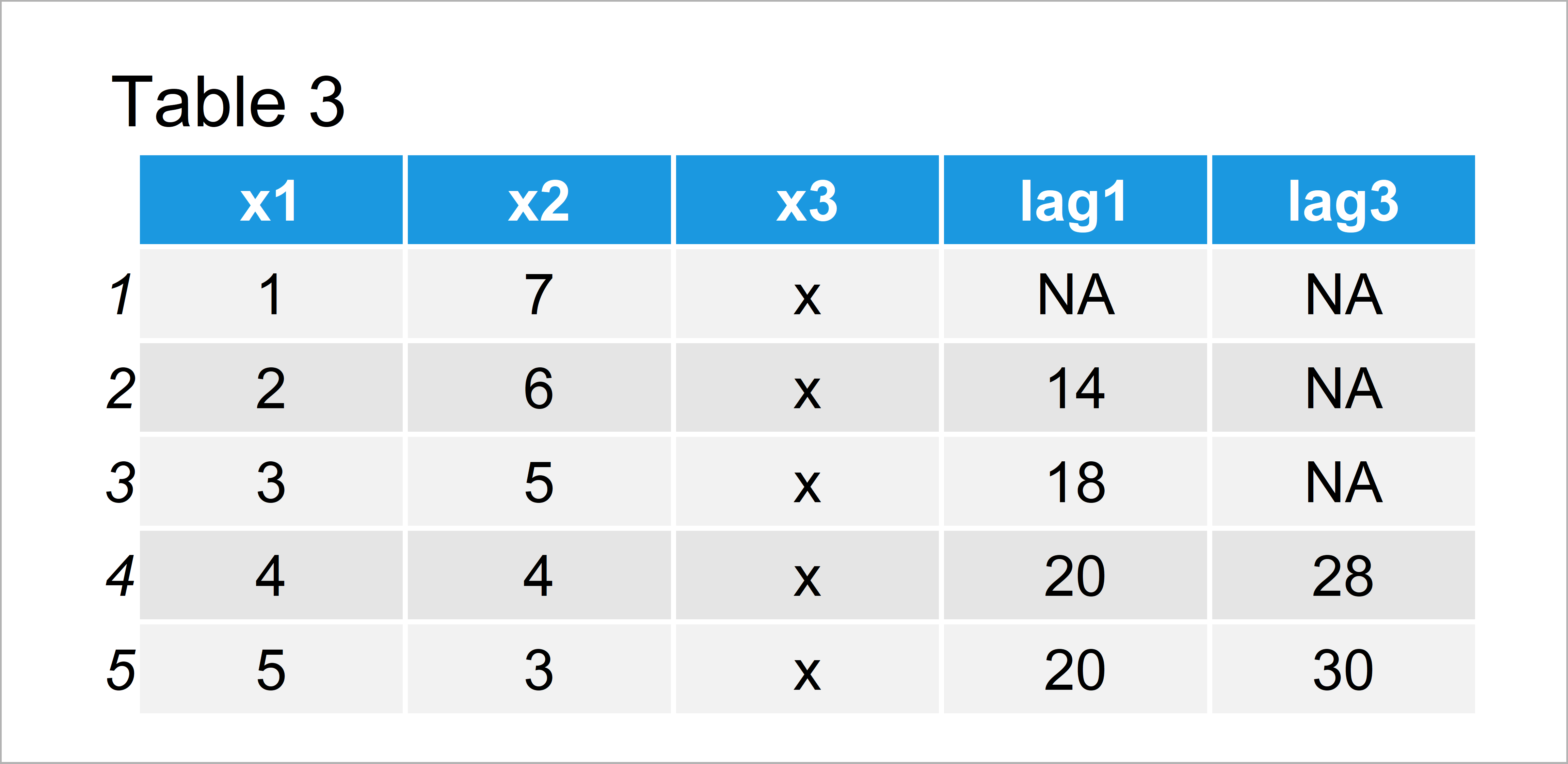# Use Previous Row of data.table in R (2 Examples)

In this article, I’ll show how to select values from the previous row of a data.table in the R programming language.

The content of the page is structured as follows:

Let’s dive right in!

## Example Data & Add-On Packages

We first have to install and load the data.table package, in order to use the functions that are contained in the add-on package.

```install.packages("data.table")                    # Install & load data.table
library("data.table")```

Next, we can create an exemplifying data.table as shown below:

```data <- data.table(x1 = 1:5,                      # Create example data.table
x2 = 7:3,
x3 = "x")
data                                              # Print example data.table```Table 1 shows that our exemplifying data.table consists of five rows and five columns.

## Example 1: Extract Previous Row from data.table Using shift() Function

This example explains how to get the values from one row before when doing calculations with a data.table.

For this task, we can use the shift function like this:

```data[ , lag1 := x1 * shift(x2)]                   # Use previous row
data                                              # Print updated data```The output of the previous R programming code is illustrated in Table 2 – We have created a new data.table column called lag1 that contains the values of the variable x1 time the values of the previous row in the variable x2.

## Example 2: Extract N Rows Before from data.table Using shift() Function & type Argument

This example explains how to extract values from earlier rows in a data.table. In this example, we’ll use the values that are three rows before the current row.

For this, we can specify the type argument within the shift function to be equal to “lag”:

```data[ , lag3 := x1 * shift(x2, 3, type = "lag")]  # Use three rows before
data                                              # Print updated data```As shown in Table 3, the previous R programming code has created a data.table with another new column called lag3.

## Video & Further Resources

Would you like to know more about data.tables? Then I can recommend watching the following video of my YouTube channel. In the video, I illustrate the R codes of this page.

Please accept YouTube cookies to play this video. By accepting you will be accessing content from YouTube, a service provided by an external third party.If you accept this notice, your choice will be saved and the page will refresh.

Also, you might have a look at the other tutorials of statisticsglobe.com. You can find a selection of articles about data manipulation and data.tables below:

This article has explained how to extract the previous row of a data.table when doing calculations in R. Please let me know in the comments section, in case you have additional questions.

Subscribe to the Statistics Globe Newsletter

•Stephen Jones
September 9, 2022 4:28 pm

I tried to simply find the difference, as follows, but it did not work?
data[ , lag1 := x1 – shift(x1)]

•September 9, 2022 8:25 pm

Hey Stephen,

Is the diff function doing what you are looking for?

Regards,
Joachim

•Stephen Jones
September 9, 2022 9:15 pm

Wouldn’t it need to be adjusted to work in a data frame?

•September 19, 2022 11:42 am

You may use Base R functions for data.tables as well. Have a look at the following example code:

```library("data.table")

data <- data.table(x1 = c(1, 3, 8, 2, 1),
x2 = 7:3,
x3 = "x")
data
#    x1 x2 x3
# 1:  1  7  x
# 2:  3  6  x
# 3:  8  5  x
# 4:  2  4  x
# 5:  1  3  x

data[ , new := c(NA, diff(x1))]
data
#    x1 x2 x3 new
# 1:  1  7  x  NA
# 2:  3  6  x   2
# 3:  8  5  x   5
# 4:  2  4  x  -6
# 5:  1  3  x  -1```

Regards,
Joachim